# Direct product of D8 and Z2

View a complete list of particular groups (this is a very huge list!)[SHOW MORE]

This particular group is a finite group of order: 16

## Definition

This group is defined in the following ways:

A presentation for it is:$G := \langle a,x,y \mid a^4 = x^2 = y^2 = e, xax = a^{-1}, ya = ay, xy = yx \rangle$.

## Elements

### Upto conjugacy

There are ten conjugacy classes:

1. The identity element. (1)
2. The element$a^2$. (1)
3. The element$y$. (1)
4. The element$a^2y$. (1)
5. The two-element conjugacy class comprising$a$ and$a^3$. (2)
6. The two-element conjugacy class comprising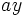$ay$ and$a^3y$. (2)
7. The two-element conjugacy class comprising$x$ and$a^2x$. (2)
8. The two-element conjugacy class comprising$xy$ and$a^2xy$. (2)
9. The two-element conjugacy class comprising$ax$ and$a^3x$. (2)
10. The two-element conjugacy class comprising$axy$ and$a^3xy$. (2)

### Upto automorphism

Under the action of the automorphism group, the conjugacy classes (3) and (4) are in the same orbit -- in other words,$y$ and$a^2y$ are related by an automorphism. The conjugacy classes (5) and (6) are equivalent, and the conjugacy classes (7)-(10) are equivalent. Thus, the equivalence classes have sizes$1,1,2,4,8$.

## Arithmetic functions

Function Value Explanation
order 16
exponent 4 Cyclic subgroup of order four in dihedral part.
nilpotency class 2
derived length 2
Frattini length 2
Fitting length 1
minimum size of generating set 3 Generating set of dihedral direct factor and generating element for other direct factor (also equal to rank of Frattini quotient).
subgroup rank 3
max-length 4
rank as p-group 3 Direct product of Klein four-subgroup of first direct factor with second direct factor.
normal rank 3 Direct product of Klein four-subgroup of first direct factor with second direct factor.
characteristic rank of a p-group 2 Center is a Klein four-subgroup, no characteristic elementary abelian subgroups of order eight

## Group properties

Property Satisfied Explanation Comment
Abelian group No$a$ and$x$ don't commute
Nilpotent group Yes Prime power order implies nilpotent
Metacyclic group No
Supersolvable group Yes Finite nilpotent implies supersolvable
Solvable group Yes Nilpotent implies solvable
T-group No$\langle x \rangle \triangleleft \langle a^2,x \rangle$, which is normal, but$\langle x \rangle$ is not normal Smallest example for normality is not transitive.
Monolithic group No Center is Klein four-group, any subgroup of order two is minimal normal.
One-headed group No Distinct maximal subgroups of order eight.
SC-group No
ACIC-group Yes Every automorph-conjugate subgroup is characteristic
Rational group Yes Any two elements that generate the same cyclic group are conjugate Thus, all characters are integer-valued.
Rational-representation group Yes All representations over characteristic zero are realized over the rationals. Contrast with quaternion group, that is rational but not rational-representation.
Directly indecomposable group No
Centrally indecomposable group No

## Subgroups

Further information: Subgroup structure of direct product of D8 and Z2

The group has the following subgroups:

1. The trivial group. (1)
2. The cyclic group$\langle a^2 \rangle$ of order two. This is central, and is also the set of squares. Isomorphic to cyclic group:Z2. (1)
3. The subgroups$\langle y \rangle$ and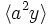$\langle a^2y \rangle$. These are both central subgroups of order two, but are related by an outer automorphism. Isomorphic to cyclic group:Z2. (2)
4. The subgroups$\langle x \rangle$,$\langle ax \rangle$,$\langle a^2x \rangle$,$\langle a^3x \rangle$,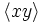$\langle xy \rangle$,$\langle axy \rangle$,$\langle a^2xy \rangle$, and$\langle a^3xy$. These are all related by automorphisms, and are all 2-subnormal subgroups. They come in four conjugacy classes, namely the class$\langle x \rangle, \langle a^2x \rangle$, the class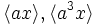$\langle ax \rangle, \langle a^3x \rangle$, the class$\langle xy \rangle, \langle a^2xy$, and the class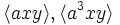$\langle axy \rangle, \langle a^3xy \rangle$. Isomorphic to cyclic group:Z2. (8)
5. The subgroup$\langle a^2, y \rangle$. This is the center, hence is a characteristic subgroup. Isomorphic to Klein four-group. (1)
6. The subgroups$\langle a^2, x \rangle$,$\langle a^2, ax \rangle$,$\langle a^2, xy \rangle$, and$\langle a^2, axy \rangle$. These are all normal subgroups, but are related by outer automorphisms. Isomorphic to Klein four-group. (4)
7. The subgroups$\langle y, x \rangle$,$\langle y, ax \rangle$,$y, a^2x$,$\langle y, a^3x$,$\langle a^2y, x \rangle$,$\langle a^2y, ax$,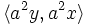$\langle a^2y, a^2x \rangle$,$\langle a^2y, a^3x \rangle$. These subgroups are all 2-subnormal subgroups and are related by outer automorphisms, and they come in four conjugacy classes of size two. Isomorphic to Klein four-group. (8)
8. The subgroups$\langle a \rangle$ and$\langle ay \rangle$. They are both normal and are related via an outer automorphism. Isomorphic to cyclic group:Z4. (2)
9. The subgroups$\langle a^2,x,y \rangle$ and$\langle a^2, ax, y \rangle$. These are normal and are related by outer automorphisms. Isomorphic to elementary abelian group of order eight. (2)
10. The subgroups$\langle a,x \rangle$,$\langle a, xy \rangle$,$\langle ay, x \rangle$ and$\langle ay, xy \rangle$. These are both normal and are related by an outer automorphism. Isomorphic to dihedral group:D8. (4)
11. The subgroup$\langle a, y \rangle$. This is a characteristic subgroup. Isomorphic to direct product of Z4 and Z2. (1)
12. The whole group. (1)

## GAP implementation

### Group ID

The ID of this group in GAP's list of groups of order$16$ is$11$. The group can be described using GAP's SmallGroup function as:

SmallGroup(16,11)

### Other descriptions

It can be described as a direct product:

DirectProduct(DihedralGroup(8),CyclicGroup(2))